# User:Tohline/Appendix/PolytropicBinaries

 |   Tiled Menu   |   Tables of Content   |  Banner Video   |  Tohline Home Page   |

# Polytropic Models of Close Binary Star Systems

Over the past half-a-dozen years, Patrick Motl, Mario D'Souza, and Wes Even have used the Hachisu SCF technique to construct 3D equilibrium models of synchronously rotating, tidally distorted binary polytropes. To date, four of these models have been used extensively as initial states for our dynamical simulations of binary mass-transfer. Various properties of these four SCF-code-generated models are summarized in the following table; the listed parameters are: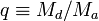$q \equiv M_d/M_a$  : System mass ratio M  : Mass a  : Binary separation Ω  : Orbital angular velocity Jtot  : Total angular momentum ρmax  : Maximum (central) density Kn  : Constant in the polytropic equation of state,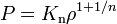$~P = K_\mathrm{n} \rho^{1+1/n}$ V  : Volume occupied by the star or by the Roche Lobe (RL) surrounding the star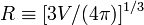$R\equiv [3V/(4\pi)]^{1/3}$  : Mean stellar radius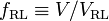$f_\mathrm{RL} \equiv V/V_\mathrm{RL}$  : Roche-lobe filling factor

 Properties of (n = 3 / 2) Polytropic Binary Systems Model Binary System Accretor Donor q Mtot a Ω Jtot Ma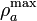$\rho^\mathrm{max}_a$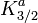$K^a_{3/2}$ Ra Md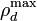$\rho^\mathrm{max}_d$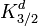$K^d_{3/2}$ Rd fRL Q13 1.323 0.0309 0.8882 0.2113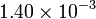$1.40\times 10^{-3}$ 0.0133 1.0000 0.0264 0.2672 0.0176 0.6000 0.0372 0.3509 0.968 Q07 0.70000 0.02371 0.83938 0.20144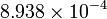$8.938\times 10^{-4}$ 0.013945 1.0000 0.02732 0.2728 0.009761 0.6077 0.02512 0.2888 0.998 Q05 0.500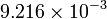$9.216\times 10^{-3}$ 0.8764 0.1174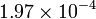$1.97\times 10^{-4}$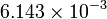$6.143\times 10^{-3}$ 1.0000 0.016 0.2067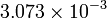$3.073\times 10^{-3}$ 0.235 0.016 0.2689 0.898 Q04 0.4085 0.02399 0.8169 0.2112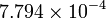$7.794\times 10^{-4}$ 0.01703 1.0000 0.03119 0.2918 0.006957 0.71 0.01904 0.2453 0.996 References: Model Q13 (q = 1.323): Table 4 in publication DMTF06 Model Q07 (q = 0.700): First page of the accompanying PDF document. NOTE: In this PDF document, Roche-lobe volumes appear to be too large by factor of 2. Model Q05 (q = 0.500): Table 5 in publication DMTF06 Model Q04 (q = 0.4085): Table 1 in publication MFTD07

All of the parameter values listed in these tables are specified in dimensionless polytropic units, defined as follows:

 Polytropic Units Here, Polytropic Units are defined such that the radial extent of the computational grid for the self-consistent-field (SCF) model, Redge, the maximum density of one binary component,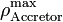$\rho^\mathrm{max}_\mathrm{Accretor}$, and the gravitational constant, G, are all unity, that is,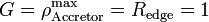$G = \rho^\mathrm{max}_\mathrm{Accretor} = R_\mathrm{edge} = 1$. In an accompanying PDF document, we explain how to convert from this set of dimension code units to real (e.g., cgs) units.

 © 2014 - 2019 by Joel E. Tohline |   H_Book Home   |   YouTube   | Context: | PGE | SR | Appendices: | Equations | Variables | References | Binary Polytropes | Ramblings | Images | Images (2016 Layout) | ADS |